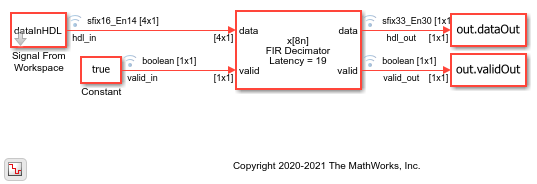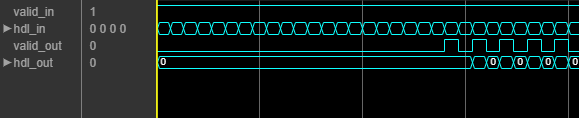# FIR Decimation for FPGA

This example shows how to decimate streaming samples using a hardware-friendly polyphase FIR filter. It also shows the differences between the `dsp.FIRDecimator` object and the hardware-friendly streaming interface of the FIR Decimator block.

Generate an input sine wave in MATLAB®. The example model imports this signal from the MATLAB workspace. Choose a decimation factor, coefficients, and the input vector size for the HDL-optimized block.

```T = 512; waveGen = dsp.SineWave(0.9,100,0,'SamplesPerFrame',T); dataIn = fi(waveGen()+0.05,1,16,14); decimFactor=8; coeffs = firpm(30,[0 0.1 0.2 0.5]*2,[1 1 0 0]); inputVecSize=4; ```

The example model imports the input signal from the MATLAB workspace and applies it as vectors to the FIR Decimator block. The model returns the output from the block to the MATLAB workspace for comparison.

The FIR Decimator block applies samples to the filter in a different phase than the `dsp.FIRDecimator object`. To match the numerical behavior, apply `decimFactor` - 1 zeros to the FIR Decimator block before the start of the data samples.

```dataInHDL = [zeros(decimFactor-1,1);dataIn]; modelName = 'FIRDecimHDL'; open_system(modelName); set_param(modelName,'SimulationCommand','Update'); ```The FIR Decimator block accepts a vector of `inputVecSize`-by-1 samples and returns scalar output. The block performs single-rate processing and indicates valid samples in the output signal by setting the output valid signal to `1`. The Simulink sample time is `inputVecSize` for the whole model. The block sets the output valid signal to `1` every `decimFactor/inputVecSize` samples.```out = sim('FIRDecimHDL'); ```

Create a `dsp.FIRDecimator` System object™ and generate reference data to compare against the HDL model output. The object can accept any input vector size that is a multiple of the decimation factor, so you can calculate the output with one call to the object. If you change the decimation factor to a value that is not a factor of `T`, you must also adjust the value of `T` (size of `dataIn`).

The block and the object are both configured to use full-precision internal data types. The object computes a different output data type in this mode. This difference means that the output might not match if the internal values saturate the data types.

```decimRef = dsp.FIRDecimator(decimFactor,'Numerator',coeffs); refDataOut = decimRef(dataIn); ```

For the output of the FIR Decimator block, select data samples where the output valid signal was `1`, and compare them with the samples returned from the `dsp.FIRDecimator` object.

```hdlVec = out.dataOut(out.validOut); refVec = refDataOut(1:size(hdlVec,1)); errVec = hdlVec - refVec; maxErr = max(abs(errVec)); fprintf('\nFIR decimator versus reference: Maximum error out of %d values is %d\n',length(hdlVec),maxErr); ```
```FIR decimator versus reference: Maximum error out of 55 values is 0 ```